# Examples of 2D Darcy flows

The following two examples come from [BD].

## 1. Example : 2D Darcy flow, chessboard pressure

### 1.1. Input parameters

Notation Quantity Type Unit

$\underline{\underline\kappa}$

Permeability

order 2 tensor

$m^2Pa^{-1}s^{-1}$

$f(x,y)$

Flux source term

scalar function

$s^{-1}$

### 1.2. Model & Toolbox

We consider a 2D unit square $\Omega=[0,1]\times[0,1]$ whose boundary is denoted $\Gamma$. The following problem is to be solved in $\Omega$.

$\left\{\begin{array}{rll} \underline u+\underline{\underline\kappa}\nabla p=&0&\text{in }\Omega\\ \nabla\cdot\underline u=&f&\text{in }\Omega\\ \end{array}\right.$

with the additional condition $\int_\Omega f=0$. The pressure is denoted $p$ and the velocity $\underline u$. We assume the material permeability is constant, isotropic and unitary, that is $\underline{\underline\kappa}=\underline{\underline{I_d}}$.

Let us define the source term $f=\nabla\cdot\underline u=-\Delta p$ to get the analytic solution $p(x,y)=sin(2\pi x)cos(2\pi y)$. It yields $f(x,y)=8\pi^2sin(2\pi x)cos(2\pi y)$.

This example runs within the Mixed Poisson toolbox with prescribed parameters.

### 1.3. Boundary conditions

We impose a Dirichlet boundary condition on the whole boundary : $p=sin(2\pi x)cos(2\pi y)\text{ on }\Gamma$.

### 1.5. Convergence analysis

Table 1. P0 convergence analysis
$h$ 0.2 0.1 0.05 0.01 0.005

$\Vert p-p_h\Vert_{L^2}$

9.57939e-01

5.42923e-01

2.78594e-01

5.6416e-02

2.83271e-02

$\Vert u-u_h\Vert_{L^2}$

2.78314e-01

1.35505e-01

6.61506e-02

1.30739e-02

6.52889e-03

Table 2. P1 convergence analysis
$h$ 0.2 0.1 0.05 0.01 0.005

$\Vert p-p_h\Vert_{L^2}$

1.69091e-01

4.85275e-02

1.26349e-02

5.14523e-04

1.28986e-04

$\Vert u-u_h\Vert_{L^2}$

1.66947e-01

4.78222e-02

1.22767e-02

4.92702e-04

1.23431e-04

Table 3. P2 convergence analysis
$h$ 0.2 0.1 0.05 0.01 0.005

$\Vert p-p_h\Vert_{L^2}$

2.22396e-02

3.15292e-03

4.07591e-04

3.22962e-06

4.0602e-07

$\Vert u-u_h\Vert_{L^2}$

1.73431e-02

2.35603e-03

3.01594e-04

2.33871e-06

2.93291e-07

Table 4. P3 convergence analysis
$h$ 0.2 0.1 0.05 0.01 0.005

$\Vert p-p_h\Vert_{L^2}$

2.03629e-03

1.52963e-04

9.81156e-06

1.56186e-08

9.80369e-10

$\Vert u-u_h\Vert_{L^2}$

1.37478e-03

1.01811e-04

6.43878e-06

1.00732e-08

6.3051e-10

### 1.6. Output

The following output example is reproducible using `feelpp_toolbox_mixed-poisson-model_2DP2` running on 12 cores with the previous `.json` and `.cfg` files on a mesh of typical size $h=0.05$.

The screenshots are in order : the pressure field, the velocity magnitude field and the velocity field.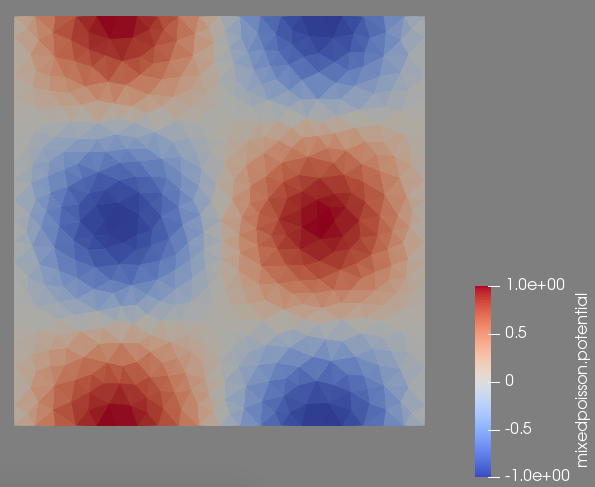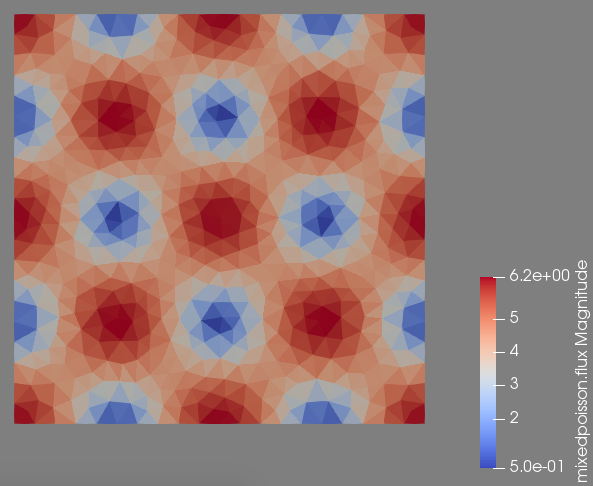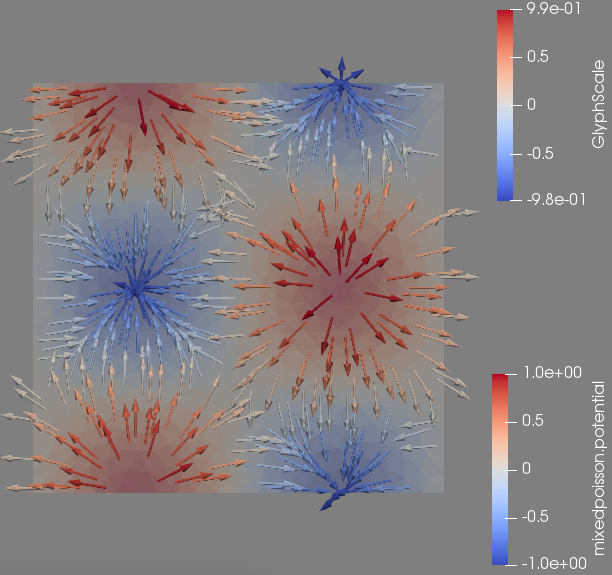### 1.7. Example : Darcy flow, shower

The input parameters, model and toolbox are the same as in the previous example.

### 1.8. Boundary conditions

We impose a Dirichlet boundary condition on the whole boundary : $p=sin(y)sin(x)+xy^2-\frac16-\sin(1)(1-\cos(1))\text{ on }\Gamma$.

### 1.10. Convergence analysis

Table 5. P0 convergence analysis
$h$ 0.2 0.1 0.05 0.01 0.005

$\Vert p-p_h\Vert_{L^2}$

5.34577e-02

2.79542e-02

1.42528e-02

2.85709e-03

1.43102e-03

$\Vert u-u_h\Vert_{L^2}$

4.78442e-02

2.43738e-02

1.23471e-02

2.45374e-03

1.22648e-03

Table 6. P1 convergence analysis
$h$ 0.2 0.1 0.05 0.01 0.005

$\Vert p-p_h\Vert_{L^2}$

1.97729e-03

5.33807e-04

1.34873e-04

5.41901e-06

1.35843e-06

$\Vert u-u_h\Vert_{L^2}$

6.14894e-03

1.61917e-03

3.99372e-04

1.52692e-05

3.81444e-06

Table 7. P2 convergence analysis
$h$ 0.2 0.1 0.05 0.01 0.005

$\Vert p-p_h\Vert_{L^2}$

1.40696e-05

1.91059e-06

2.46414e-07

1.95803e-09

2.45484e-10

$\Vert u-u_h\Vert_{L^2}$

5.16536e-05

7.12397e-06

9.13825e-07

7.16198e-09

8.98457e-10

Table 8. P3 convergence analysis
$h$ 0.2 0.1 0.05 0.01 0.005

$\Vert p-p_h\Vert_{L^2}$

2.47985e-07

1.81459e-08

1.16742e-09

1.80373e-12

2.89473e-13

$\Vert u-u_h\Vert_{L^2}$

6.13595e-07

4.34515e-08

2.77315e-09

4.19972e-12

1.17702e-12

### 1.11. Output

The following output example is reproducible using `feelpp_toolbox_mixed-poisson-model_2DP2` running on 12 cores with the previous `.json` and `.cfg` files on a mesh of typical size $h=0.05$.

The screenshots are in order : the pressure field, the velocity magnitude field and the velocity field.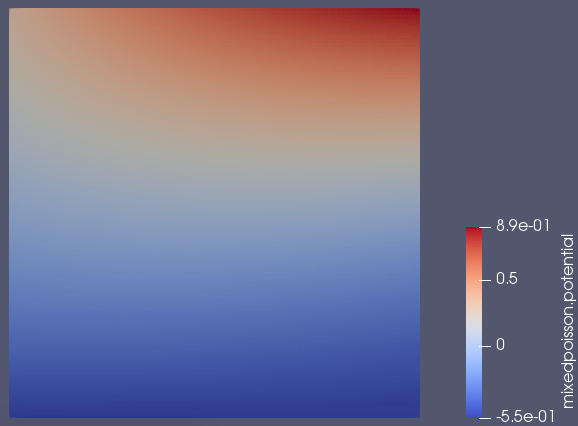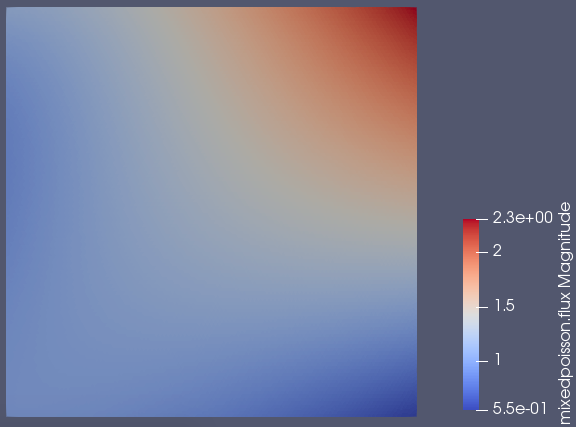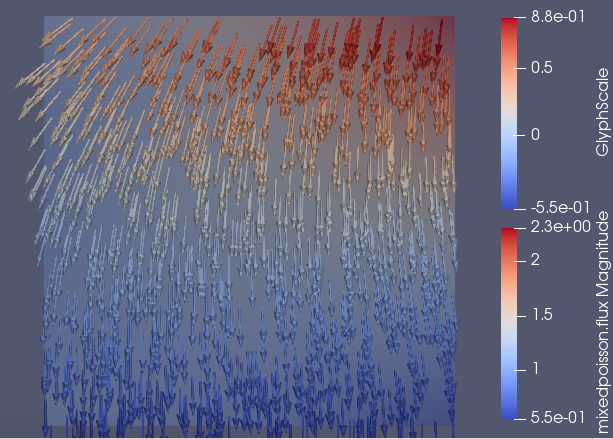### 1.12. Reference

[BD] Pavel B. Bochev, Clark R. Dohrmann, A computational study of stabilized, low-order $C^0$ finite element approximations of Darcy equations